Zhang, S., Huang, Z., Wu, Y., and Zhu, Y. (2023). "Effect of thermal bridge in light-frame wood wall," BioResources 18(1), 367-381.

#### Abstract

The presence of thermal bridges in a wall increases local heat conduction of the building envelope, resulting in a decrease in the wall’s average thermal resistance. Simultaneously, the internal surface temperature of thermal bridge is lower than that of the surrounding areas and shows a tendency of condensation. Therefore, it is necessary to employ thermal bridges in the stage of construction design. In the research, a two-dimensional steady-state numerical simulation was performed targeting thermal bridges with light-frame wood wall. Meanwhile, the heat bridge effect was simulated under different circumstances by changing the types of insulation and cladding materials, the number of the studs, and the framing factor. The results showed that the linear heat transfer coefficient increased linearly as the studs and framing factor rose. After the test was validated, the relative error rate between the simulated correction coefficients and the experimentally derived correction coefficients was 11.4%, indicating that the correction coefficients can be simulated.

Effect of Thermal Bridge in Light-Frame Wood Wall

Siyi Zhang,* Zilan Huang, Yue Wu, and Yixin Zhu

The presence of thermal bridges in a wall increases local heat conduction of the building envelope, resulting in a decrease in the wall’s average thermal resistance. Simultaneously, the internal surface temperature of thermal bridge is lower than that of the surrounding areas and shows a tendency of condensation. Therefore, it is necessary to employ thermal bridges in the stage of construction design. In the research, a two-dimensional steady-state numerical simulation was performed targeting thermal bridges with light-frame wood wall. Meanwhile, the heat bridge effect was simulated under different circumstances by changing the types of insulation and cladding materials, the number of the studs, and the framing factor. The results showed that the linear heat transfer coefficient increased linearly as the studs and framing factor rose. After the test was validated, the relative error rate between the simulated correction coefficients and the experimentally derived correction coefficients was 11.4%, indicating that the correction coefficients can be simulated.

DOI: 10.15376/biores.18.1.367-381

Keywords: Light-frame wood wall; Linear heat transfer coefficient; Simulation; Thermal bridge; Correction coefficient

Contact information: College of Materials Science and Engineering, Nanjing Forestry University, Nanjing, 210037, China; *Corresponding author: zsy@njfu.edu.cn

INTRODUCTION

In the context of global environmental degradation and increasing pressure of the energy crisis, low-energy wooden buildings have broad prospects. The presence of thermal bridges is one of the main factors affecting the energy consumption (Choi et al. 2022).

Thermal bridges created by the discontinuity of thermal insulations as parts of the building envelope have major effects on the thermal performance. The influence of thermal bridges can amount to 30% relative to the heating needs (Capozzoli et al. 2013). The study of Bjarløv showed that there are a lot of thermal bridges in the roof, the façades, and the floor, all of which led to heat losses (Bjarløv and Vladykova 2011).

Thermal bridges in building envelopes should be taken as a priority to improve the thermal performance of buildings, because they increase heat loss in buildings and reduce the temperature of the internal envelope surface, causing moisture condensation and mold breeding (Torres-Rivas et al. 2018). Given the definition of ISO 10211 (2017), the places where the building envelop is penetrated by a more thermally conductive material, where the thickness of the structure changes, or where there is a difference in internal and external surface area of the joints such as walls and floors, all these places that meet one of these criteria is called a thermal bridge.

Both the simulation and experiment techniques have been analyzed in various ways aiming at understanding thermal bridges. A series of calculations are applied, according to some methods. The effect of thermal bridges is expressed by linear thermal transmittance (Capozzoli et al. 2013; O’Grady et al. 2017), which can be calculated relying on relevant standards. Since the complexity of two- or three-dimensional dynamic heat transfer simulation has led to a dilemma in practical operation, the steady-state method has been utilized in most studies on the thermal behavior of construction elements (Viot et al. 2015). Building energy simulation programs, such as TRNSYS, THERM, and WUFI, are commonly used to analyze the effect of thermal bridge in different conditions and predict the behavior and the energy consumption of buildings (Martin et al. 2011, 2012; De Angelis and Serra 2014; Levinskyte et al. 2016). Simulation of a realistic linear thermal bridge by assuming a dynamic behaviour is achieved by employing boundary element simulation (BEM) to control the dynamics of the internal and external temperatures at the thermal bridge (Tadeu et al. 2011). An approach based on the equivalent wall method is adopted to replace the thermal bridge by defining a one-dimensional three-layer wall. The comparison between this approach and the existing four methods has shown that this approach can obtain more accurate results (Quinten and Feldheim 2016). Therefore, the experimental results are used to validate the simulation predictions obtained with software (Zalewski et al. 2010; Cuce and Cuce 2016).

For wooden buildings, some works have been published on the characterization of the thermal behavior, static or dynamic, of linear thermal bridges to energy performance of buildings. The PSIPLAN software was utilized by Ligia (Moga and Moga 2015) to analyze the thermal bridge in different insulation conditions of light frame wood wall. The software Flixo was applied by Pusila (2015) to conduct the simulation, and the results showed that the thermal bridge in cross-laminated timber CLT buildings eradiated less heat than that in concrete buildings. Additionally, hot box was used by Joana (Prata et al. 2018) to analyze the heat transfer at the corner of the CLT wall and the experimental results were compared with the simulation results, showing a good agreement. Seong (Chang et al. 2019) studied the heat transfer of the wall with ply-laminate cross-laminated timber (ply-lam CLT) by experiment and simulation, which is a brand-new structure. The results indicate that the ply-lam CLT walls have lower thermal bridges than wood frame structure walls.

In this paper, a combination of experiment and simulation was used to study the repetitive thermal bridges in light-frame wood walls. The linear heat transfer coefficient was used as a method to analyze the effect of thermal bridge quantitatively, while the change of linear heat transfer coefficient can reflect the degree of influence of thermal bridges on heat transfer in walls. Finally, the relative error between the simulated correction coefficients and the experimentally derived correction coefficients can be used to verify the accuracy of the simulation.

EXPERIMENTAL

Materials

The materials are shown in Fig. 1. The stud used spray polyurethane foam (SPF) that was made in Canada with the specification being 2” × 4”. The cladding panel used oriented strand board (OSB) with the specification being 1220 mm × 2440 mm, the thickness being 12 mm, and the apparent density being 690 kg/cm3. The gypsum board was used in the inner wall panel with the specification being 1220 mm × 2440 mm, the thickness being 12 mm, and the areal density being 9.5 kg/m2. The insulation material was made of glass wool with the bulk density being 480 kg/cm3.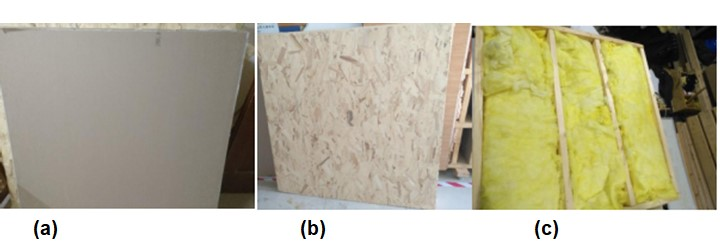Fig. 1. Experimental materials: (a) gypsum board; (b) OSB; and (c) glass wool

Methods

In the test, the temperature control box-heat flow meter method was utilized, which provided a constant temperature difference through the cold and hot boxes to achieve a stable heat transfer state of the wall. Moreover, the temperature was used to measure the temperature of the cold and hot surfaces of the wall, and the heat flux density sensor was used to test the heat flux density through the wall. After the test wall reached stable heat transfer, several sets of data were selected for analysis and the heat transfer coefficient was calculated.

The main instruments in this experiment consisted of a JIRJ-B heat transfer coefficient intelligent detector, heat box with temperature controller, twenty temperature sensors, two heat flow meters, etc. All these instruments were purchased from Zhejiang Jinhua Julong Testing Machine Co., Ltd. (Jinhua, China). The temperature sensors could measure the temperature from -50 to 150 °C with an accuracy of ± 0.5 °C, a resolution of 0.1 °C, and a heat flow coefficient of 8 J/(m2⋅s). This measurement of the heat transfer coefficient was in accordance with GB/T23483 (2009) and GB 50176 (2016).

As for the distribution points of the light frame wood wall, there were three measurement points at the insulation, six measurement points at the stud, and one heat flow sensor at the stud and insulation, respectively. The wall to be tested was fixed with a cold box and heat box, and the place of connection between the upper and lower boxes and the wall was wrapped with insulation to prevent the inaccurate results caused by a large amount of heat leakage during the test. The temperature of the hot box was set at 40 °C, so that the temperature difference between inside and outside remained at 20 °C, and the temperature difference with the simulation was guaranteed to be the same. The instrument was preheated first, during which the changes in temperature and heat flow were observed. After the wall’s heat transfer reached a stable state, the temperature change was less than 1% and the data were recorded.

According to GB/T 23483 (2009), the thermal resistance was calculated with Eq. 1,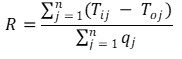(1)

where is the thermal resistance of the building envelop to be tested [(m2·K)/W], Tij is the j-th measured value of the inner surface temperature of the building envelop (°C), is the j-th measured value of the outer surface temperature of the building envelop (°C), and Qj is the j-th measured value of the heat flux density of the building envelop (W/m2). The heat transfer coefficient of the tested wall can be calculated by Eq. 2,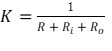(2)

where R is the thermal resistance to be tested ((m2·K)/W), Ri and Ro are both the heat transfer resistances inside and outside of the wall surface, 0.11 (m2·K/W) and 0.043 (m2·K/W) respectively, and K is the heat transfer coefficient of the wall to be tested [W/(m2·K)].

Simulation

The calculation unit

Each stud of the wood-frame construction wall was considered the same, and one stud was extracted as a calculation unit for analysis, while the effect of nails had been ignored in the study. Figure 2 shows a schematic diagram of the calculation unit model with the size of the stud as 2” × 4”.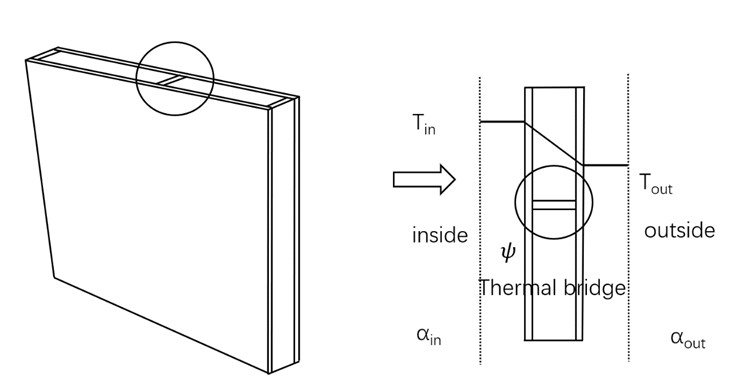Fig. 2. Thermal bridge computing unit model diagram

Simulation variables

The repetitive thermal bridge exists at the stud of the wood-frame wall, which is an unavoidable thermal bridge phenomenon and may be related to the type of insulation materials, cladding materials, the number of the studs and framing factor, etc. Therefore, the effect was analyzed in this paper from the following aspects.

(1) Insulation materials

The stud of the wood-frame wall was filled with insulation materials to increase the thermal performance. Three types of insulation materials are selected, and the specific parameters are listed in Table 1.

Table 1. Thermophysical Parameters of Insulation Materials (China Architecture and Building Press 2010)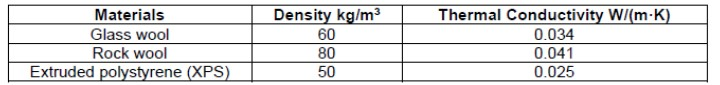In this study, three kinds of cladding materials are selected for simulation, and the specific parameters are presented in Table 2.

Table 2. Thermophysical Parameters of Cladding Materials (China Architecture and Building Press 2010)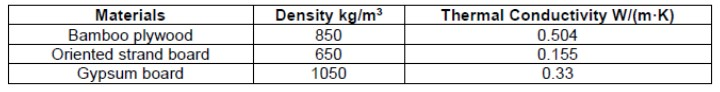(3) The number of wood studs

The typical stud size of 2” × 4” of the wall was selected, and the number of studs was changed. In addition, the proportion of the wall occupied by studs remained unchanged. When the simulation of one stud was conducted, the stud area was assumed as c and the wall area was assumed as ab, and thereby the proportion of the wall occupied by stud area was c/ab. Similarly, as the simulation of three studs was performed, the stud area was 3c, and the wall area was 3ab, and the proportion occupied remained the same. And so on, the proportion occupied by 5 and 7 studs was unchanged. The model is shown schematically in Fig. 3.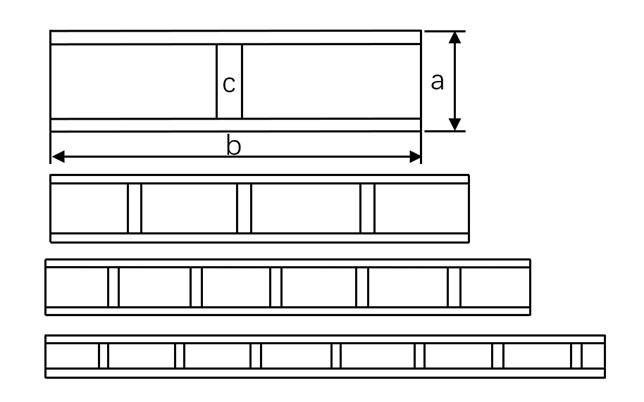Fig. 3. The model of the stud numbers

(4) Framing factor

In current practical tests and theoretical analysis of building envelopes, the number of members incorporated into the total flat wall area often refers to the framing factor, which is equal to the frame’s percentage to the entire wall area (Syed and Kośny 2006). As an important consideration for light-frame wood wall, in this study, the wall width was set to a fixed value of 406 mm, and the stud width was the product of the wall width and the framing factor, which was changed by varying the framing factor (Christensen 2011). The dimension is illustrated in Table 3.

Table 3. The Model Size of Framing Factor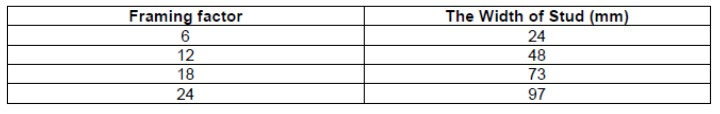Calculation of linear heat transfer coefficient in simulation

According to the ISO 10211 (2017), the influence of building thermal bridge on heat transfer is described, and the detailed calculation and simulation methods are given. The following is the calculation formula of the heat transfer coefficient of the thermal bridge: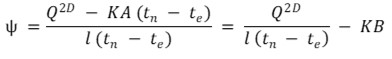(3)

In this equation, the is the linear heat transfer coefficient of thermal bridge (W/(m·K)), Q2D is the heat flow through the wall (W), is the heat transfer coefficient of the main section of the wall [W/(m2·K)], is the area of the rectangular wall for calculating the heat flow (m2), is the length of one side of the wall, and the thermal bridges are evenly distributed along this length, usually 1 m in the calculation, tn is the air temperature inside the wall (°C), te is the air temperature outside the wall (°C), and B is the length of the other side of the rectangular wall (m).

Given that the influence of temperature difference on the linear heat transfer coefficient had not been considered, the indoor and outdoor temperature difference was set to 20 °C. According to the GB 50176 (2016), the outdoor temperature (Tout) was set to 0 °C, the indoor temperature (Tin) was 20 °C, the inside surface coefficient of heat transfer αin was 8.7 W/(m2·K), and the outside surface coefficient of heat transfer αout was 23 W/(m2·K).

Calculation of the mean heat transfer coefficient of the test wall

The mean heat transfer coefficient in the test of the wood-frame construction wall was calculated by the weighted area method according to the Eq. 4.

K = Kw · Sw + Kc · Sc (4)

In Eq. 4, the K is the mean heat transfer coefficient of the test wall (W/(m2·K)), KW is the heat transfer coefficient of the thermal insulation (W/(m2·K)), and w is the proportion of the thermal insulation area, at 93% in this test. The KC is the heat transfer coefficient of stud [W/(m2·K)], and Sc is the proportion of the stud area, 7%.

Coefficient correction of heat transfer coefficient

According to JGJ26-2018 (2018), the correction coefficient of the heat transfer coefficient can be calculated by Eqs. 5 and 6.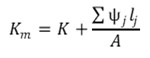(5)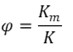(6)

As shown in the above equations, the Km is the average heat transfer coefficient of the unit wall [W/(m2·K)], K is the heat transfer coefficient of the main section of the unit wall [W/(m2·K)], lj is the line heat transfer coefficient of the j-th thermal bridge on the unit wall(m), A is the area of the unit wall (m2), and φ is the correction coefficient of the heat transfer coefficient of the main section of the unit wall [W/(m·K)].

RESULTS AND DISCUSSION

Validation

The measurements of the heat transfer coefficient were according to GB/T23483 (2009) and GB 50176 (2016). The test results are illustrated in Fig. 4.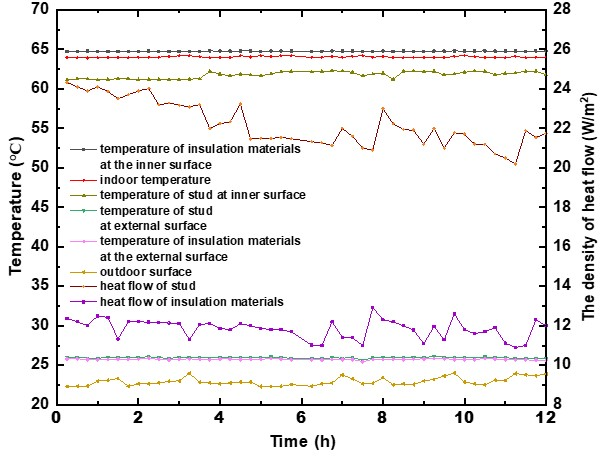Fig. 4. Test results of light-frame wood wall

The temperature on the inner surface of the studs in the light-frame wood wall was 3 ~ 4 °C lower than that on the inner surface of the insulation materials. The heat flux density of the studs in the light-frame wood wall ranged from 20.35 to 24.33 W/m2, and the heat flux density of the insulation ranged from 10.08 to 12.92 W/m2. It can be stated that the change of heat flux density at the thermal bridge in the light frame wood wall was more drastic than that at the non-thermal bridge, because the thermal bridge constructed a heat path that was more inclined to transfer through the thermal bridge. Compared with the non-thermal bridge, the thermal bridge amassed more heat, which also caused the heat flux density to be more obvious. It is essential to analysis the influence of the thermal bridge and mitigate the heat loss. The test results of light-frame wood wall were recorded, and the thermal resistance and the heat transfer coefficient were also calculated according to Eqs. (1), (2), and (4). The results are listed in Table 4.

Table 4. Test Values of Heat Transfer Coefficient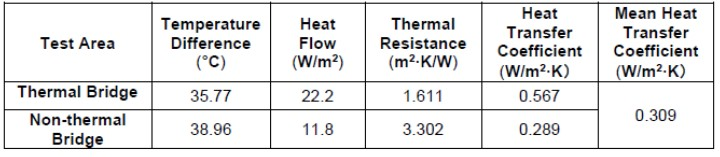Effect of Insulation Materials on Linear Heat Transfer Coefficient

In the case of the same framing factor, cladding materials, and number of studs, the changes of the linear heat transfer coefficients of three types of insulation materials, glass wool, rock wool, and XPS, were simulated under the stud sizes of 2” × 4”, 2” × 6”, and 2” × 8” respectively. Figure 4 presents the linear heat transfer coefficients which are expressed based on these simulation values regarding Eq. 3.

Figure 5 shows that under the same insulation materials, the size of the stud increased from 2” × 4” to 2” × 8”, and the linear heat transfer coefficient decreased obviously. And when the stud size changed from 2” × 4” to 2” × 8”, the slope was almost unchanged, it can be seen that the changing trends was mainly affected by the stud.

When the size of the stud remained unchanged, for different insulation materials, the variation of linear heat transfer coefficient was less than 0.001 (W/(m.k)), which suggested that the effect of insulation materials on the linear heat transfer coefficient was slight. Moreover, the linear heat transfer coefficient of the wall with rock wool was highest, which indicated that the insulation material with higher thermal conductivity has a more distinct effect from the thermal bridge.

Effect of Cladding Materials on Linear Heat Transfer Coefficient

Under the condition that the framing factor, the insulation materials, and the number of studs were consistent, the stud of 2” × 4” was selected as a typical size to simulate three cladding materials with different thicknesses. According to the simulation results in Fig. 6, the values decreased with the increase of the thickness. When the thickness remained unchanged, the linear heat transfer coefficient of bamboo plywood was higher than that of gypsum board and OSB. It can be noted that although the thermal conductivity of the gypsum board was about two times higher than that of OSB, the linear heat transfer coefficient of gypsum board was not much higher than that of OSB at the same thickness Similarly, the thermal conductivity of bamboo plywood was bigger than that of OSB and gypsum board, but the difference of linear heat transfer coefficient obtained by simulation was small. Therefore, the impact of cladding materials on the linear heat transfer coefficient was much smaller than the difference between the thermal conductivity of cladding materials.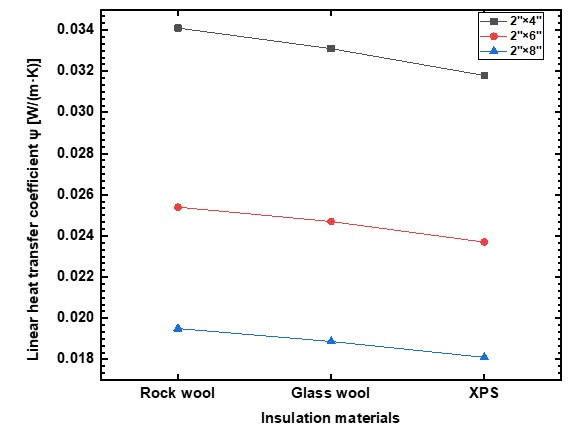Fig. 5. Linear heat transfer coefficient diagram of different insulation materials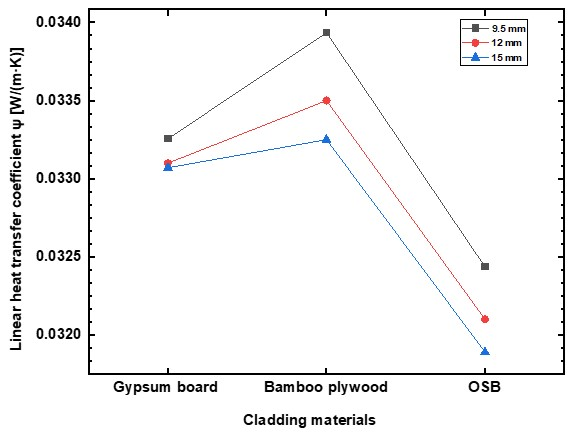Fig. 6. Linear thermal transmittance coefficient diagram of different cladding materials

Effect of the Number of Studs on the Linear Heat Transfer Coefficient

Under the condition that the framing factor, the insulation materials, and the cladding materials were consistent, the stud of 2” × 4” was selected, the linear heat transfer coefficient of the wall was simulated when the number of studs was 1, 3, 5, and 7 respectively.

Figure 7 shows a schematic diagram indicating the linear heat transfer coefficient of the wall with different stud quantities. The estimated value was figured out based on the calculation value of one stud multiplied by the corresponding stud number. The almost linear increase of the linear heat transfer coefficient indicated that the number of studs had a more considerable impact on the results. The reason was mainly attributed to the fact that the increase of the number of studs drives the growth of the corresponding nodes forming thermal bridges, thus causing an upward trend in the linear heat transfer coefficient.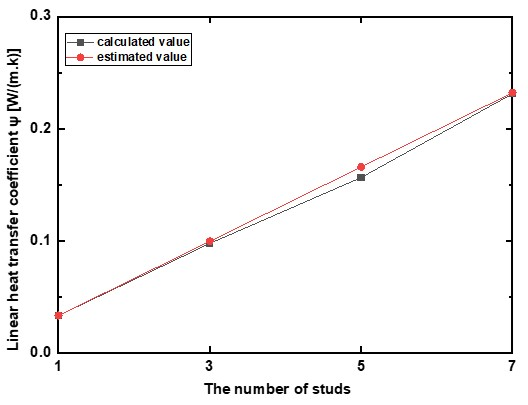Fig. 7. Linear heat transfer coefficient of different number studs

The rounding of the decimal places of the linear heat transfer coefficient also influenced the results, so it needed to be considered. As illustrated in Table 5, the calculated and estimated values of the linear heat transfer coefficient were almost equal within the allowable error range. Therefore, the linear heat transfer coefficient for different stud numbers can be calculated through an estimation without the necessity of building a complete model.

Table 5. Influence of the Decimal Places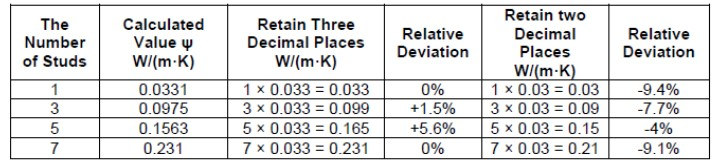Effect of Framing Factor on Linear Heat Transfer Coefficient

Under the condition that the other variables were the same, the light-frame wood wall’s linear heat transfer coefficients with framing factors of 6%, 12%, 18%, 24%, and 30% were simulated, respectively. According to Fig. 8, the value was augmented linearly with the increase of framing factor, indicating that the framing factor had a considerable influence on the results.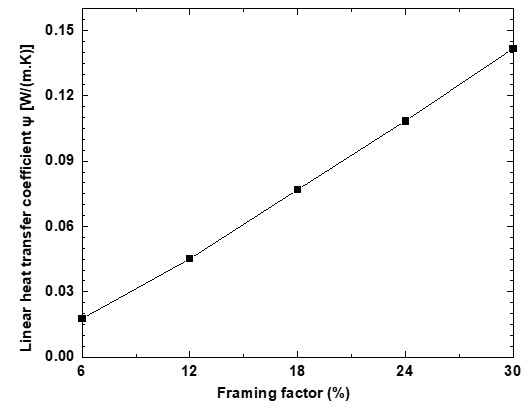Fig. 8. Linear heat transfer coefficient of different framing factor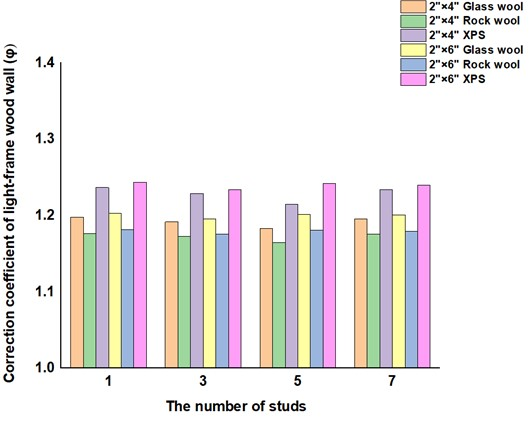Fig. 9. Correction coefficient of light-frame wood wall

Correction Coefficient of Heat Transfer Coefficient of Light-Frame Wood Wall

In line with the above analysis, the insulation materials, the stud’s size, the number of studs, and the framing factor had all been considered in terms of the wall’s correction coefficient. Figure 9 demonstrates the correction coefficient of the light-frame wood wall with different factors. On the pretext of ensuring the other variables to be the same, a different number of studs had little influence on the correction coefficient, which could be ignored.

It can be noted that the curve changed linearly, as shown in Fig. 9. When the number of the studs remained unchanged and the framing factor became larger, so as the correction coefficient. Additionally, the change of the difference in correction coefficient was in keeping with that of the framing factor. By linearly fitting the values in Fig. 9, the relationship between the correction coefficient and the framing factor f is shown in Table 5.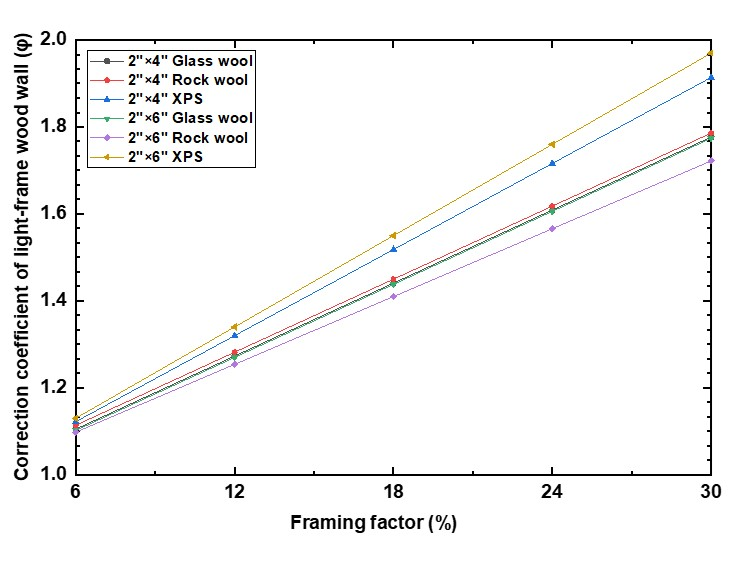Fig. 10. Correction coefficient under different framing factors

Table 6. Relationship Between Correction Coefficient and Framing Factor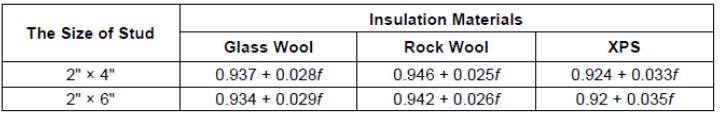According to Tables 4 and 6, the simulation correction coefficient and the test correction coefficient of the test wall were obtained and recorded in Table 7. Through the calculation of the relative errors, it can be seen that the test correction and simulation correction coefficient were 1.066 and 1.189, respectively, and the relative error rate was 11.6%, which was relatively small and could be regarded as within the error range. The error may be due to the use of nail connections in the fabrication of the specimen, and the thermal conductivity of the nails leads to an increase in heat transfer during the test, which is not considered in the model calculation.

Table 7. Comparison of Experimental and Simulated Values of Correction Coefficient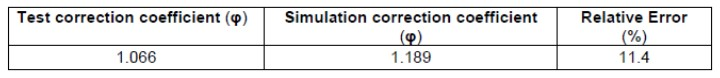CONCLUSIONS

Thermal bridges are known to influence the thermal performance of a wall. The originality of this study pertains to the special case of the light-frame wood wall. In this case, the linear heat transfer coefficient was implemented to qualify the influence of thermal bridge in different circumstances. The correction coefficient was proposed to analyze the accuracy of simulation. It is an effective way when considering how to mitigate the influence of thermal bridge in light-frame wood wall, thus rendering good performance of the wall. The results are as follows:

1. The linear heat transfer coefficient of light-frame wood wall decreased with the increase of thickness. The lower the thermal conductivity of the insulation material, the lower the linear heat transfer coefficient. The influence of different cladding materials on the linear heat transfer coefficient of the wall was relatively small, and the linear heat transfer coefficient increased linearly with the increase of the number of studs and the framing factor, thus strengthening the thermal bridge’s influence.
2. The coefficient of determination of the heat transfer coefficient which was mainly influenced by the insulation materials, the framing factor, and the wall thickness, was slightly related to the number of studs. The relative error rate between the experimental and simulated values of the correction coefficient was 11.4% through the wall’s thermal test, which indicated that the method of using software to simulate the correction coefficient was feasible.

ACKNOWLEDGMENTS

This research was supported by the planning subject of the “Twelfth Five-Year-Plan” in the National Science and Technology for the Rural Development in China: “Integration and Demonstration of Green Improvement Technology for Traditional Wooden Farm Houses” (Grant No. 2015BAL03B03-03).

REFERENCES CITED

Bjarløv, S. P., and Vladykova, P. (2011). “The potential and need for energy saving in standard family detached and semi-detached wooden houses in arctic Greenland,” Building and Environment 46, 1525-1536. DOI:10.1016/j.buildenv.2010.12.004

Capozzoli, A., Gorrino, A., and Corrado, V. (2013). “A building thermal bridges sensitivity analysis,” Applied Energy 107, 229-243. DOI: 10.1016/j.apenergy.2013.02.045

Chang, S. J., Wi, S., and Kim, S. (2019). “Thermal bridging analysis of connections in cross-laminated timber buildings based on ISO 10211,” Construction and Building Materials 213, 709-722. DOI: 10.1016/j.conbuildmat.2019.04.009

China Architecture and Building Press (2010). Handbook of Thermophysical Properties and Data of Building Materials, Institute of Building Physics, Chinese Academy of Building Sciences, Beijing, China.

Choi, J., Kim, C., Jang, H., and Kim, E. (2022). “Dynamic thermal bridge evaluation of window-wall joints using a model-based thermography method,” Case Studies in Thermal Engineering 35, article 102117, DOI: 10.1016/j.csite.2022.102117

Christensen, D. (2011). Thermal Impact of Fasteners in High-Performance Wood-Framed Walls (NREL/CP-5500-47678), National Renewable Energy Laboratory, Golden, CO, USA.

Cuce, E., and Cuce, P. M. (2016). “The impact of internal aerogel retrofitting on the thermal bridges of residential buildings: An experimental and statistical research,” Energy and Buildings 116, 449-454. DOI: 10.1016/j.enbuild.2016.01.033

De Angelis, E., and Serra, E. (2014). “Light steel-frame walls: Thermal insulation performances and thermal bridges,” Energy Procedia 45, 362-371. DOI: 10.1016/j.egypro.2014.01.039

GB 50176 (2016). “Code for thermal design of civil building,” Standardization Administration of China, Beijing, China.

GB/T 23483 (2009). “Test standard for overall heat transfer coefficient of building envelope and heat supply for space heating,” Standardization Administration of China, Beijing, China.

ISO 10211 (2017). “Thermal bridges in building construction – Heat flows and surface temperatures – Detailed calculations,” International Organization for Standardization, Geneva, Switzerland.

JCJ26-2018 (2018). “Design standard for energy efficiency of residential buildings in sever colds and cold zones,” Standardization Administration of China, Beijing, China.

Levinskyte, A., Banionis, K., and Geleziunas, V. (2016). “The influence of thermal bridges for buildings energy consumption of “A” energy efficiency class,” Journal of Sustainable Architecture & Civil Engineering 15(2), 47-58. DOI: 10.5755/j01.sace.15.2.15351

Martin, K., Erkoreka, A., Flores, I., Odriozola, M., and Sala, J. M. (2011). “Problems in the calculation of thermal bridges in dynamic conditions,” Energy and Buildings 43(2-3), 529-535. DOI: 10.1016/j.enbuild.2010.10.018

Martin, K., Escudero, C., Erkoreka, A., Flores, I., and Sala, J. M. (2012). “Equivalent wall method for dynamic characterisation of thermal bridges,” Energy and Buildings 55, 704-714. DOI: 10.1016/j.enbuild.2012.08.024

Moga, L., and Moga, I. (2015). “Thermal bridges at wood frame construction,” Journal of Applied Engineering Sciences 5(2), article 193. DOI: 10.1515/jaes-2015-0023

O’Grady, M., Lechowska, A. A., and Harte, A. M. (2017). “Infrared thermography technique as an in-situ method of assessing heat loss through thermal bridging,” Energy and Buildings 135, 20-32. DOI: 10.1016/j.enbuild.2016.11.039

Prata, J., Simões, N., and Tadeu, A. (2018). “Heat transfer measurements of a linear thermal bridge in a wooden building corner,” Energy and Buildings 158, 194-208. DOI: 10.1016/j.enbuild.2017.09.073

Pusila, J. (2015). Thermal Bridge Comparison: Thermal Benefits of CLT, Bachelor’s Thesis, Lahti University of Applied Sciences, Lahti, Finland.

Quinten, J., and Feldheim, V. (2016). “Dynamic modelling of multidimensional thermal bridges in building envelopes: Review of existing methods, application and new mixed method,” Energy and Buildings 110, 284-293. DOI: 10.1016/j.enbuild.2015.11.003

Syed, A. M., and Kośny, J. (2006). “Effect of framing factor on clear wall R-value for wood and steel framed walls,” Journal of Building Physics 30(2), 163-180. DOI: 10.1177/1744259106068884

Tadeu, A., Simões, I., Simões, N., and Prata, J. (2011). “Simulation of dynamic linear thermal bridges using a boundary element method model in the frequency domain,” Energy and Buildings 43(12), 3685-3695. DOI: 10.1016/j.enbuild.2011.10.001

Torres-Rivas, A., Palumbo, M., Haddad, A., Cabeza, L. F., Jiménez, L. and Boer, D. (2018). “Multi-objective optimisation of bio-based thermal insulation materials in

building envelopes considering condensation risk,” Applied Energy 224, 602-614. DOI: 10.1016/j.apenergy.2018.04.079

Article submitted: November 18, 2021; Peer review completed: February 26, 2022; Revised version received and accepted: November 1, 2022; Published: November 15, 2022.

DOI: 10.15376/biores.18.1.367-381